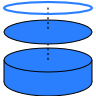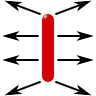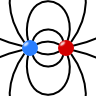Charges and their Electric Fields

Gauss's law $$\nabla\cdot\mathbf{E}\left(\mathbf{r}\right) = \rho\left(\mathbf{r}\right)/\varepsilon_{0}$$ relates the electric field to charges. Learn the basic techniques to determine the electric field $$\mathbf{E}\left(\mathbf{r}\right)$$ and/or the electrostatic potential $$\phi\left(\mathbf{r}\right)$$ for given charge distributions $$\rho\left(\mathbf{r}\right)$$!

The Homogeneously Charged Disk: Electrostatic Potential and Electric FieldTo have a certain solution at hand is often useful construct another one out of it. This is the case for the electrostatic potential and field of the charged ring that can be generalized to the homogeneously charged disk and cylinder. Find out how to calculate the solutions on the axis of symmetry.

The Electric Field and Potential of a Homogeneously Charged SphereTo find the electrostatic potential of a general charge distribution can be quite complicated. However, if symmetries are present, the problem can be boiled down to the solution of much simple(r) equation(s). Let us employ the spherical symmetry of the homogeneously charged sphere to determine its potential and electric field!

The Electric Field of a Point ChargeIf we would make a poll about the most fundamental question of electrostatics, the field of a point charge is very likely the winner. You may already know the answer but are you able to derive it directly from Maxwell's equations?

A Line Charge: Electrostatic Potential and FieldOne of the fundamental charge distributions for which an analytical expression of the electric field can be found is that of a line charge of finite length. Nevertheless, the result we will encounter is hard to follow. Two limiting cases will help us understand the basic features of the result.

A Charge inside a Metallic ShellA charge close to a metal induces surface charges. But what happens if one puts some charge inside of a metallic shell? What will happen to the field in- and outside of this cavity? Also find out what how the shell screens the inner structure of an arbitrary charge distribution.

The Electric Field of two Point ChargesSometimes it happens that a thing is more than the sum of its parts. What about two charges? Can their respective electric field behave fundamentally different in some way than just a single charge? In this problem you will learn about two main concepts in electromagnetics - the superposition principle and the dipole.